# IB DP Physics: HL复习笔记9.1.3 Calculating Energy Changes in SHM

### Calculating Energy Changes in SHM

• There are many equations to learn in the topic of simple harmonic motion
• The key equations are summarised below: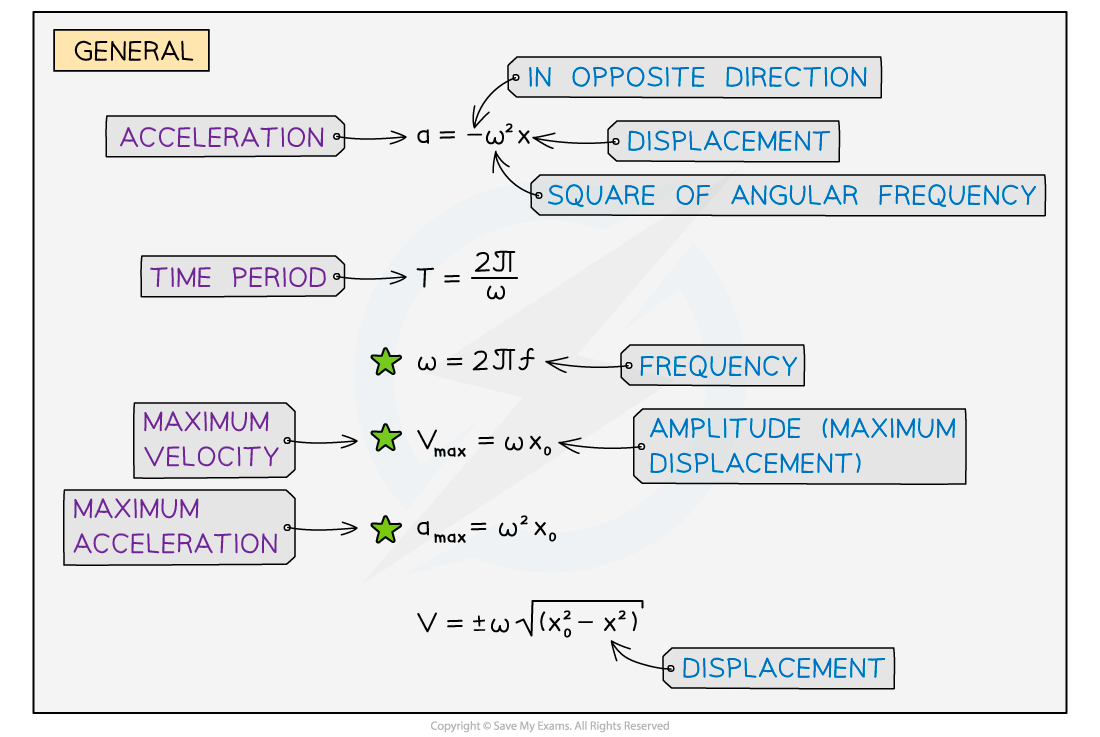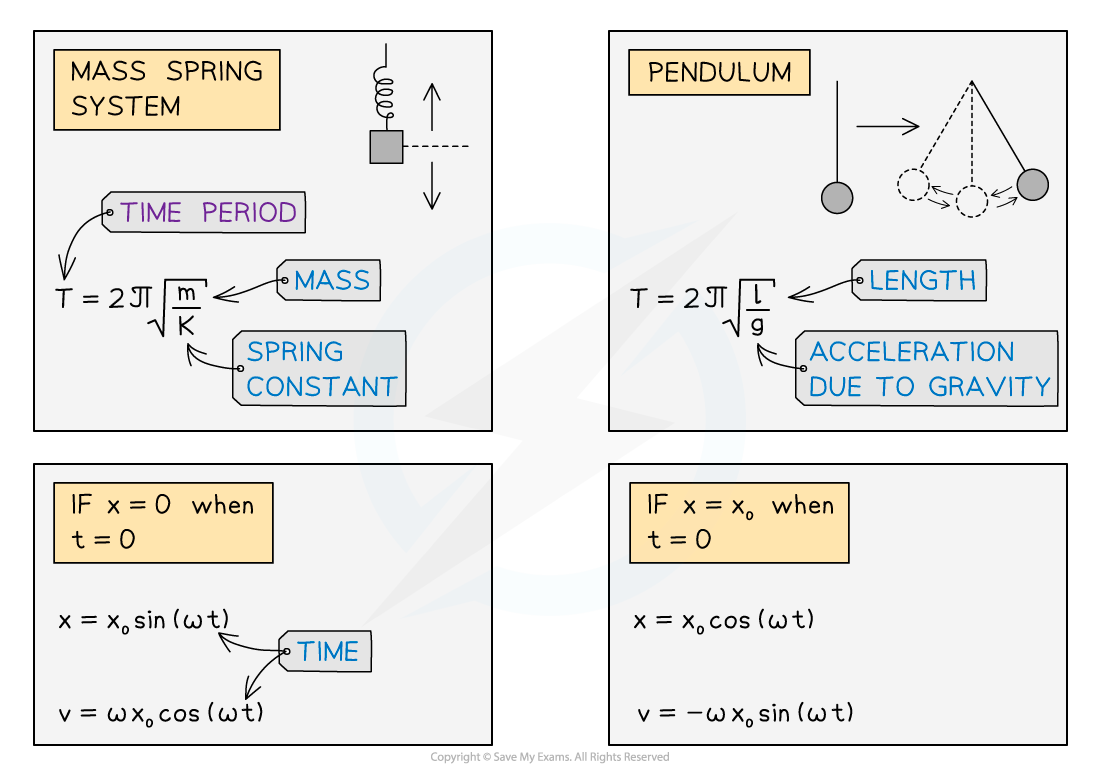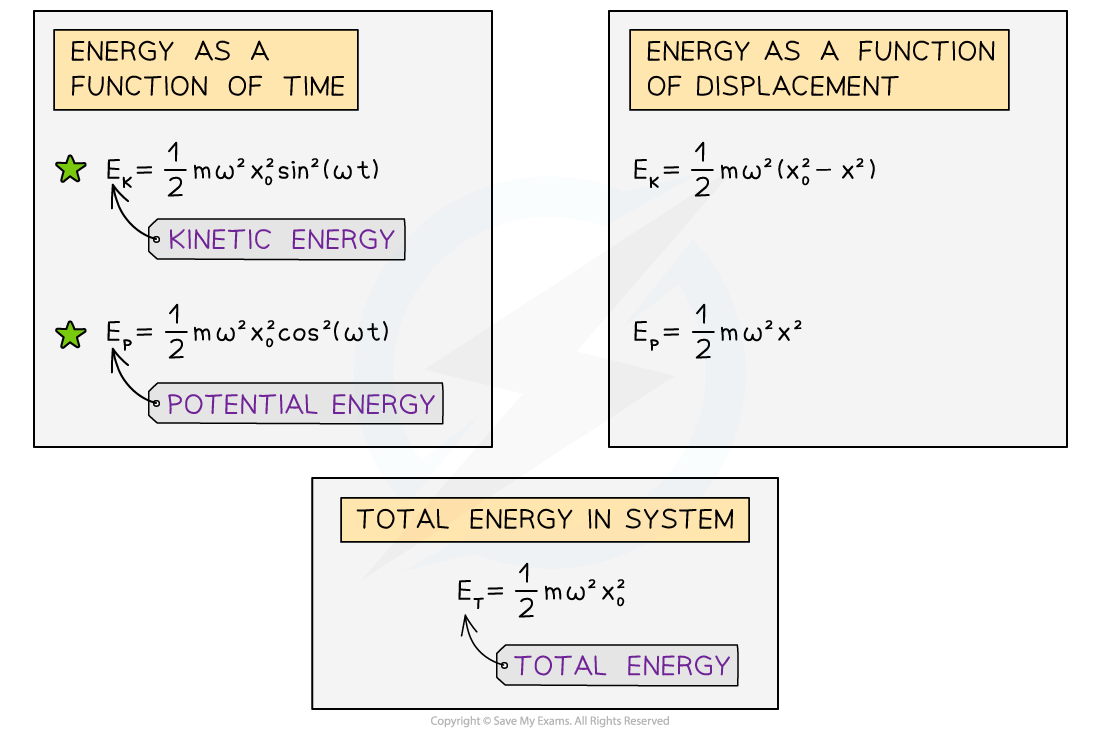A summary of the equations related to simple harmonic motion. The green stars indicate equations which are not included in the IB data booklet

#### Worked Example

The graph shows the potential energy, EP, for a particle oscillating with SHM. The particle has mass 45 g.(a)Use the graph to determine the amplitude and hence, the period of oscillation.
(b)Calculate the maximum speed which the particle achieves.

Part (a)

Step 1: List the known quantities

• Mass of the particle, m = 45 g = 45 × 10−3 kg

Step 2: Use the graph to determine the maximum potential energy of the particle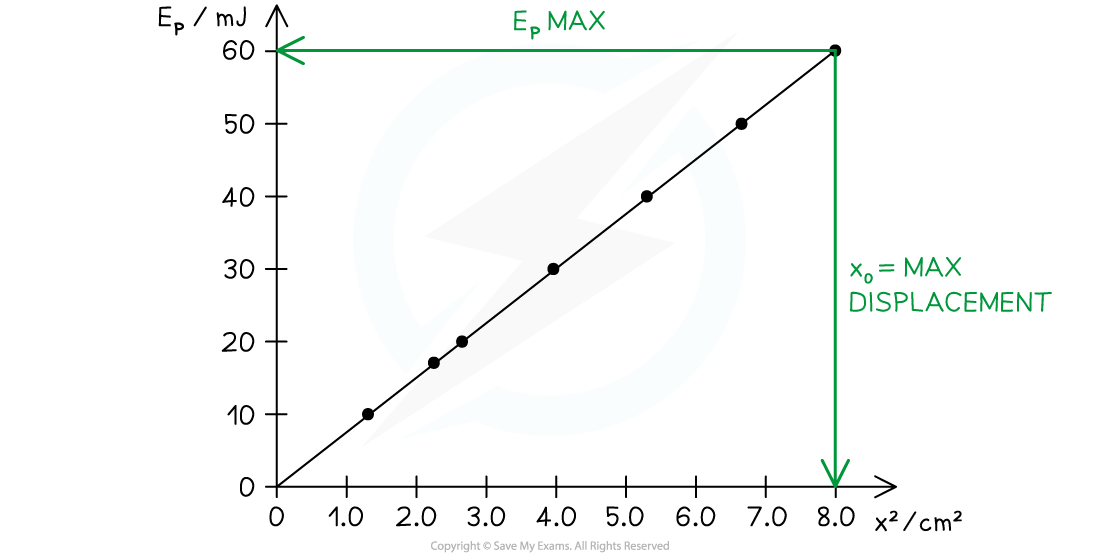• Maximum potential energy, EPmax = 60 mJ = 60 × 10−3 J

Step 3: Determine the amplitude of the oscillation

• The amplitude of the motion, x0, is the maximum displacement
• At the maximum displacement, the particle is at its highest point, hence this is the position of maximum potential energy
• From the graph, when EP = EPmax:

x02 = 8.0 cm = 0.08 m

• Therefore, the amplitude is:

x0 = √0.08 = 0.28 m

Step 4: Write down the equation for the potential energy of an oscillator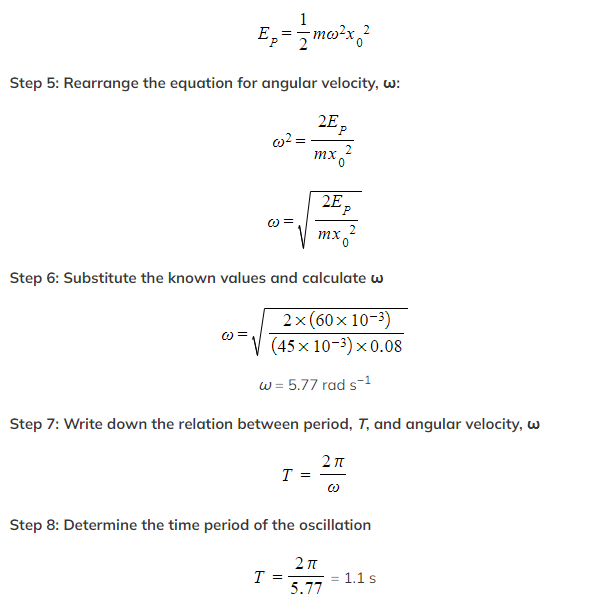Part (b)

Step 1: List the known quantities

• Angular velocity, ω = 5.77 rad s−1
• Amplitude, x0 = 0.28 m

Step 2: Write down the equation for the maximum speed of an oscillator

vmax = ωx0

Step 3: Substitute in the known quantities

vmax = 5.77 × 0.28 = 1.6 m s−1

#### Worked Example

A student investigated the behaviour of a 200 g mass oscillating on a spring, and produced the graph shown.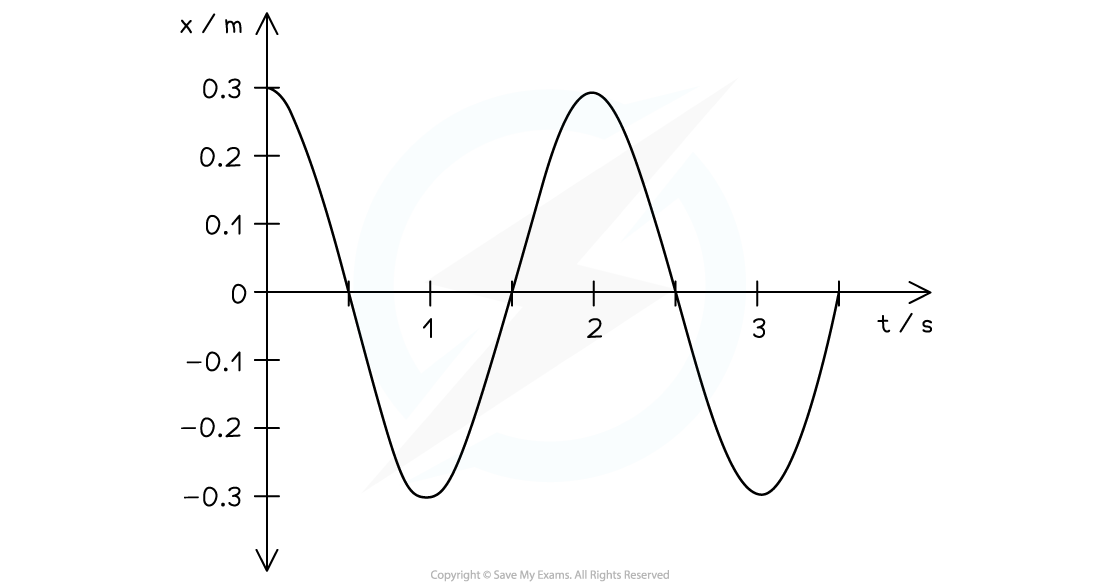(a)Determine the values for amplitude and time period
(b)Hence find the maximum kinetic energy of the oscillating mass.

Part (a)

Step 1: Read the values of amplitude and time period from the graphStep 2: State the values of amplitude and time period

• Amplitude, x0 = 0.3 m
• Time period, T = 2.0 s

Part (b)

Step 1: List the known quantities

• Mass of the oscillator, m = 200 g = 0.2 kg

Step 2: Write down the equation for the maximum speed of an oscillator

vmax = ωx0

Step 3: Write down the equation relating angular speed and time period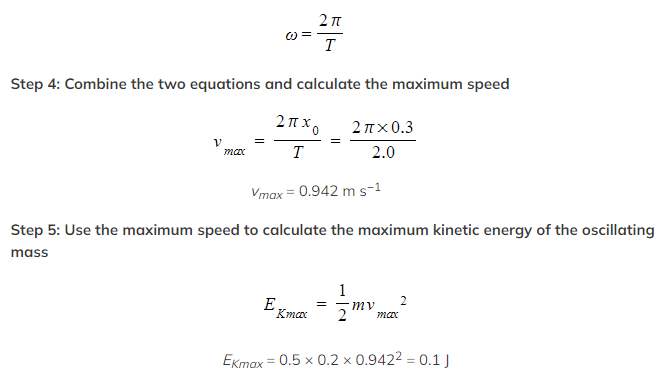#### Worked Example

A ball of mass 23 g is held between two fixed points A and B by two stretch helical springs, as shown in the diagram below.The ball oscillates along the line AB with simple harmonic motion of frequency 4.8 Hz and amplitude 1.5 cm.

Calculate the total energy of the oscillations.

Step 1: Write down all known quantities

• Mass, m = 23 g = 23 × 10–3 kg
• Amplitude, x0 = 1.5 cm = 0.015 m
• Frequency, f = 4.8 Hz

Step 2: Write down the equation for the total energy of SHM oscillations: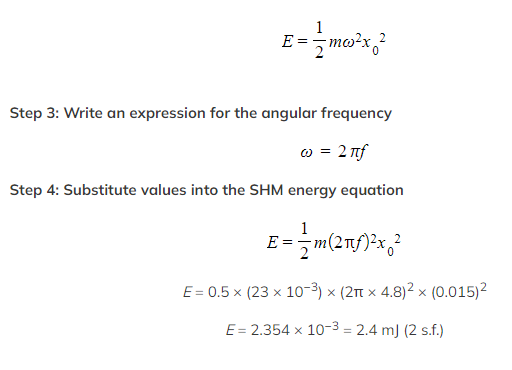#### Exam Tip

There are a large number of equations associated with SHM. Most of them are given in the data booklet which you will be given to use in the exam

Make sure you are familiar with the equations, as you will probably need to use several different ones to solve the longer questions.